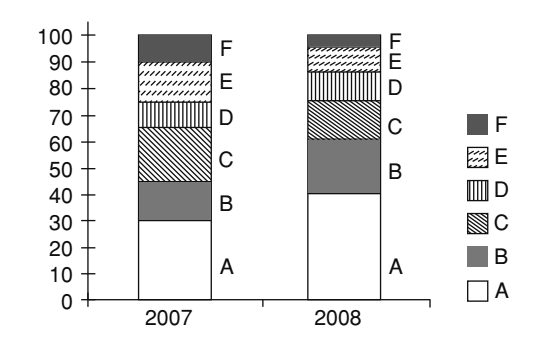## Bar graph

#### Data Interpretation

Direction: The bar chart given below shows the % distribution of the production of various models of a mobile manufacturing company in 2007 and 2008. The total production in 2007 was 35 Lakh mobile phones and in 2008 the production was 44 Lakhs.
Study the chart and answer the following questions. % of 6 different types of mobiles manufactured by a company over 2 years.1. What was the difference in the number of B type mobiles produced in 2007 and 2008?

1. Required difference = The number of B type mobiles produced in 2007 - The number of B type mobiles produced in 2008

##### Correct Option: A

Given:- The total production of mobile phones in 2007 = 35 Lakh
The total production in 2008 = 44 Lakh
Percentage of B type mobiles produced in 2007 = 20%
Percentage of B type mobiles produced in 2008 = 15%
Required difference = The number of B type mobiles produced in 2007 - The number of B type mobiles produced in 2008
Required difference = ( The total production in 2008 x Percentage of B type mobiles produced in 2007 ) - ( The total production of mobile phones in 2007 x Percentage of B type mobiles produced in 2008 )
= 44 x 20% - 35 x 15%

 = 44 x 20 - 35 x 15 100 100

 = 880 - 525 = 355 Million = 355000 100 100

1. If 85% of the D type mobiles produced in each year were sold by the company, how many D type mobiles remained unsold?

1. Required answer = The total production of mobile phones in 2007 of 10% of 15% + The total production in 2008 of 10% of 15%

##### Correct Option: C

Given:- The total production of mobile phones in 2007 = 35 Lakh
The total production in 2008 = 44 Lakh
If 85% of the D type mobiles are sold , so 15% remain unsold.
Required answer = The total production of mobile phones in 2007 of 10% of 15% + The total production in 2008 of 10% of 15%

 =35 x 10 x 15 + 44 x 10 x 15Million 100 100 100 100

 =150 x 79= 1.1850 Million 10000

=118500

1. For which models was the percentage variation in production from 2007 to 2008 the maximum?

1. In Model ,
Percentage Variation

 = Total increase x 100% Production in 2008

##### Correct Option: D

As per given table , we can see that
For A , Production in 2007 = 40 , Production in 2008 = 30
Total increase = Production in 2007 - Production in 2008
= 40 - 30 = 10
In Model ,
Percentage Variation

 = Total increase x 100% Production in 2008

 Model A ⇒ 10 x 100 = 33 1 30 3

Simillarly , For B , Production in 2007 = 20 , Production in 2008 = 15
Total increase = Production in 2007 - Production in 2008
= 20 - 15 = 5
 Model B ⇒ 5 x 100 = 33 1 15 3

For C , Production in 2007 = 15 , Production in 2008 = 20
Total increase = Production in 2007 - Production in 2008
= 15 - 20 = -5
 Model C ⇒ -5 x 100 = - 25 20

Hence required answer will be A and B models.

1. If the percentage production of A type mobiles in 2008 was same as that in 2007, then the number of A type mobiles produced in 2008 would have been

1. Required Production = The total production in 2008 x % 0f production of A type mobiles in 2007

##### Correct Option: B

Given:- The total production in 2008 = 44 Lakh
Percentage of production of A type mobiles in 2007 = 30%
Required Production = Percentage of production of A type mobiles in 2007 x The total production in 2008
= 30% of 44

 Required Production = 44 x 30 = 13,20,000 Million 100

1. Total number of mobiles of models A, B and E manufactured in 2007 was

1. Required answer = Number of mobiles of models A manufactured in 2007 + Number of mobiles of models B manufactured in 2007 + Number of mobiles of models E manufactured in 2007

##### Correct Option: C

Given:- The total production of mobile phones in 2007 = 35 Lakh
Percentage of A type mobiles produced in 2007 = 30%
Percentage of B type mobiles produced in 2007 = 15%
Percentage of E type mobiles produced in 2007 = 15%
Required answer = Number of mobiles of models A manufactured in 2007 + Number of mobiles of models B manufactured in 2007 + Number of mobiles of models E manufactured in 2007
Required answer = 30% of The total production of mobile phones in 2007 + 15% of The total production of mobile phones in 2007 + 15% of The total production of mobile phones in 2007

 = 35 x 30 + 35 x 15 + 35 x 15 100 100 100

 = 35 (30 + 15 + 15) = 35 x 60 = 21 Million 100 100